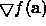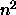Next: Quasi-Newton (Variable Metric) Up: Theory: Optimization Methods Previous: Conjugate Directions

## Multi-Dimensional Search (With First Derivatives)

Suppose that we can efficiently calculate the gradient vectorat a point (by `efficiently' we mean analytically, requiring less computation than n individual evaluations of the original function). There are cunning `Conjugate Gradient' algorithms available which use this information to build up conjugate sets much faster than Powell's method (n line minimizations rather than). See .

Bob Fisher
Fri Mar 28 14:12:50 GMT 1997